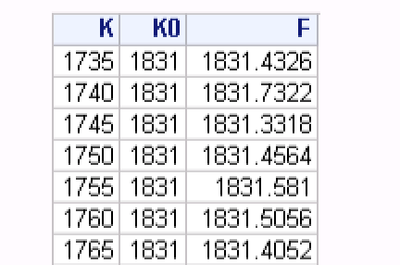## Add a label column as a vector or matrix based on condition

Hi all,

I have 3 data vectors read in iml which areI want to mark these data by following discipline:

• label = put where k<k0;
• label = call where k>k0;
• label = mix where k=k0.

How can I do? Thank you!

1 ACCEPTED SOLUTION

Accepted Solutions

## Re: Add a label column as a vector or matrix based on condition

You can use essentially the same syntax to construct Q like this:

``````  lab = choose(k<k0,'put','mix');
lab = choose(k>k0,'call',lab);
Q = choose(k<k0, p, (p+c)/2);
Q = choose(k>k0, c, Q);
print k0 k lab p c Q;``````
3 REPLIES 3

## Re: Add a label column as a vector or matrix based on condition

I think this will work

``````  lab = choose(k<k0,'put','mix');
lab = choose(k>k0,'call',lab);
print k0 k lab;``````

however you may need to screen missing values out first if you have them.

## Re: Add a label column as a vector or matrix based on condition

Awesome, it works, thanks!
however, how can I use this info to define another row? like
create a new vector Q
Q=p (a numeric variable ) if lab='put';
Q=c (a numeric variable ) if lab='call';
Q=(p+c)/2 if lab='mix';
The vector should be continuous, like
p c lab Q
1 2 put 1
2 3 call 3
4 5 call 5
6 7 mix 6.5

Can you help?

## Re: Add a label column as a vector or matrix based on condition

You can use essentially the same syntax to construct Q like this:

``````  lab = choose(k<k0,'put','mix');
lab = choose(k>k0,'call',lab);
Q = choose(k<k0, p, (p+c)/2);
Q = choose(k>k0, c, Q);
print k0 k lab p c Q;``````
From The DO Loop